# Irrational equations

## The concept of the irrational equation

Definition: an Irrational equation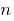is an equation containing the variable under the root signof the first degree.

## Solution of irrational equations

1. With the rise of both parts of the equation are irrational to one extent
2. In the presentation of both parts of the equation to the degree is odd (1,3,5,7....) the resulting equation is equivalent to a given ( DHS)

### Example 1:

Rozwarte equation: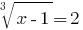Solutions: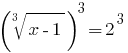Answer:In the presentation of both sides of the pair degree in (2,4,6,8....) can appear extraneous roots, which will eliminate checking.

### Example 2:

Rozwarte equation:Solutions: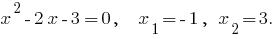Check: When you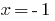haveis not a correct equation, therefore,is an extraneous root.

If youhave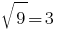- a true equality, therefore,is the root of the given equation.

Answer: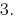3. Using the change of variables
4. If in the equation variable occurs in the same form, suchno the corresponding expression with a variable to mark one letter (a new variable).

### Example 3:

Rozwarte equation:Solution: Let Us Denote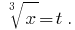Then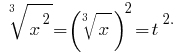We obtain the equation: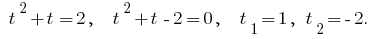Reverse the substitution: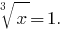Then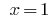or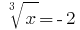here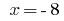.

If youhave- a true equality, therefore,is the root of the given equation.

Answer: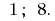Tags:
Chapter:
Versions in other languages:
Share with friends: